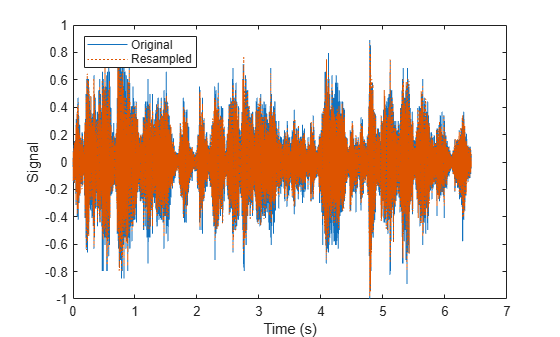partition

Partition signal datastore and return partitioned portion

Description

example

subsds = partition(sds,numPartitions,index) partitions the signal datastore into the number of parts specified by numPartitions and returns the partition corresponding to index.

example

subsds = partition(sds,'Observations',index) partitions the signal datastore and returns the partition corresponding to the index in the Observations property.

• If sds contains file data, the function partitions the signal datastore by files.

• If sds contains in-memory data, the function partitions the signal datastore by members.

subsds = partition(sds,'Observations',obsname) partitions the signal datastore and returns the partition corresponding to the observation name obsname.

• If sds contains file data, the function partitions the datastore by files.

• If sds contains in-memory data, the function partitions the datastore by members.

Examples

collapse all

Specify the file path to the example signals included with MATLAB®. Create a signal datastore that points to the specified folder.

folder = fullfile(matlabroot,'toolbox','matlab','audiovideo');
sds = signalDatastore(folder,'SampleRateVariableName','Fs');

Get the default number of partitions for the signal datastore.

n = numpartitions(sds)
n = 7

Partition the datastore into the default number of partitions and return the datastore corresponding to the fourth partition.

subsds = partition(sds,n,4);

Use the extractAfter function to display the name of the file contained in the datastore corresponding to the fourth partition.

fName = extractAfter(subsds.Files,'audiovideo\')
fName = 1x1 cell array
{0x0 char}

Read the data and information about the signal in the datastore corresponding to the fourth partition. Extract the sample rate from info and resample the signal to half the original sample rate. Plot the original and resampled signals.

while hasdata(subsds)
fs = info.SampleRate;
f_res = 0.5*fs;
ts = (0:length(data)-1)/fs;
data_res = resample(data,1,2);
t_res = (0:length(data_res)-1)/f_res;
plot(ts,data,t_res,data_res,':')
xlabel('Time (s)')
ylabel('Signal')
legend('Original','Resampled','Location','NorthWest')
endSpecify the path to a directory containing example signals included with MATLAB®.

folder = fullfile(matlabroot,'toolbox','matlab','audiovideo');

Create a signal datastore that points to the specified folder.

sds = signalDatastore(folder);

Return an estimate for a reasonable number of partitions for parallel processing, given the current parallel pool.

pool = gcp;
Starting parallel pool (parpool) using the 'local' profile ...
Connected to the parallel pool (number of workers: 6).
n = numpartitions(sds,pool)
n = 7

Partition the signal datastore and read the signal data in each part.

parfor ii = 1:n
subds = partition(sds,n,ii);
while hasdata(subds)
end
end

Input Arguments

collapse all

Signal datastore, specified as a signalDatastore object.

Number of partitions, specified as a positive integer. Use the numpartitions (Audio Toolbox) function to estimate a reasonable value for numPartitions.

Data Types: single | double

Index of sub-datastore, specified as a positive integer in the range [1,numPartitions].

Data Types: single | double

Observation name, specified as a string scalar or a character vector.

The value of obsname is:

• A file name in the case of file data.

• A member name in the case of in-memory data.

Data Types: char | string

Output Arguments

collapse all

Output signal datastore, returned as a signalDatastore object.